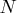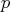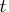Previous Page | Next Page

 Module Library

## Modules for Multivariate Random Sampling

For certain kinds of statistical simulations and Bayesian analyses, it is necessary to generate random samples ofobservations from multivariate distributions invariables. SAS/IML software provides the RANDGEN function for generating random samples from univariate distributions. However, the only subroutine for sampling from multivariate distributions is the VNORMAL call, which samples from multivariate normal distributions.

The typical method of generating a multivariate sample is to transform a sample from a related univariate distribution. Thus SAS/IML is a natural choice for generating samples from common multivariate distributions.

The SAS/IML function modules and associated multivariate distributions are as follows:

RANDDIRICHLET
generates a random sample from a Dirichlet distribution (a multivariate generalization of the beta distribution).
RANDMULTINOMIAL
generates a random sample from a multinomial distribution (a multivariate generalization of the binomial distribution).
RANDMVT
generates a random sample from a multivariate Student'sdistribution.
RANDNORMAL
generates a random sample from a multivariate normal distribution.
RANDWISHART
generates a random sample from a Wishart distribution (a multivariate generalization of the gamma distribution).

All of the modules compute their results by using transformations of univariate random samples generated by the RANDGEN function. Thus you can use the RANDSEED subroutine to set the seed for the modules.

While you can currently sample from a multivariate normal distribution by using the built-in SAS/IML subroutine VNORMAL, VNORMAL does not use the random number seed set in RANDSEED. Thus, to ensure independence and reproducibility of random number streams, the RANDNORMAL function is provided in this package.

For an overview of multivariate sampling, see Gentle (2003).Previous Page | Next Page | Top of Page